# Cauchy Schwarz inequality

Jump to: navigation, search

The Cauchy inequality for finite sums of real numbers is the inequality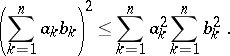Proved by A.L. Cauchy (1821); the analogue for integrals is known as the Bunyakovskii inequality.

The Cauchy inequality is also the name used for an inequality for the modulus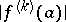of a derivative of a regular analytic function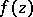at a fixed point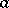of the complex plane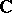, or for the modulus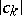of the coefficients of the power series expansion of,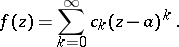These inequalities are(*)

whereis the radius of any disc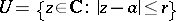on whichis regular, and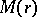is the maximum modulus of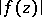on the circle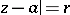. The inequalities (*) occur in the work of A.L. Cauchy (see e.g. ). They directly imply the Cauchy–Hadamard inequality (see ):where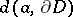is the distance fromto the boundaryof the domain of holomorphy of. In particular, ifis an entire function, then at any point,For a holomorphic functionof several complex variables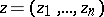,, the Cauchy inequalities are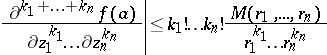orwhere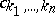are the coefficients of the power series expansion of: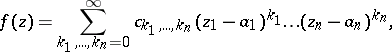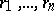are the radii of a polydisc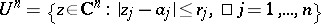on whichis holomorphic, and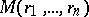is the maximum ofon the distinguished boundary of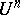.

For references see Cauchy–Hadamard theorem.

#### Comments

In Western literature the name Bunyakovskii inequality is rarely used. Both the inequality for finite sums of real numbers, or its generalization to complex numbers (see Bunyakovskii inequality), and its analogue for integrals are often called the Schwarz inequality or the Cauchy–Schwarz inequality.

The distinguished boundary of a polydiscas above is the set.

How to Cite This Entry:
Cauchy Schwarz inequality. Encyclopedia of Mathematics. URL: http://www.encyclopediaofmath.org/index.php?title=Cauchy_Schwarz_inequality&oldid=28863
This article was adapted from an original article by E.D. Solomentsev (originator), which appeared in Encyclopedia of Mathematics - ISBN 1402006098. See original article